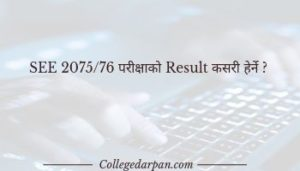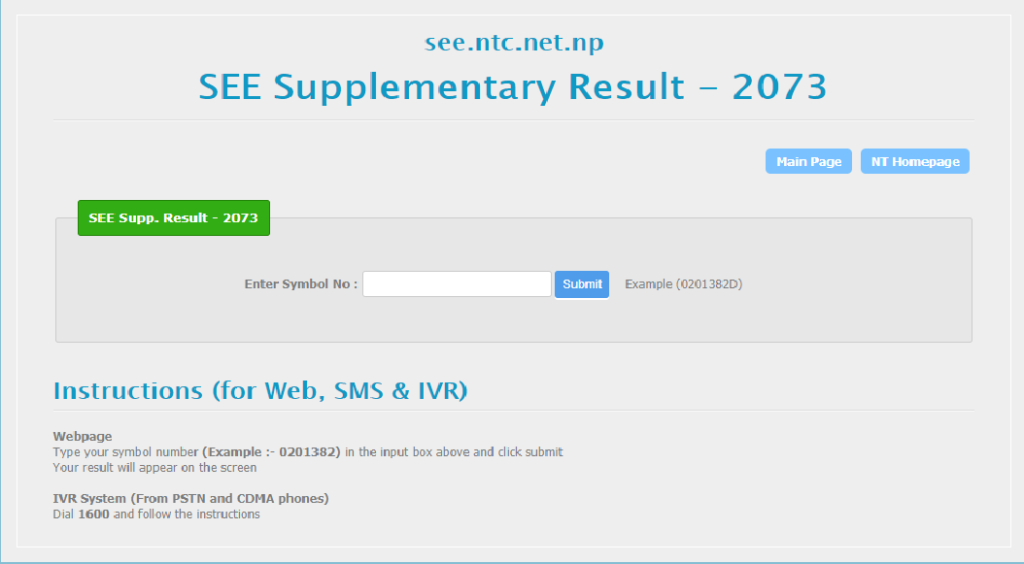S.E.E(Secondary Education Examination) formerly known as S.L.C(School Leaving Certificate) is the final examination of the secondary school system in Nepal.

Also, Check:

What after SEE exams?

Why science after SEE?# Methods to check SEE result :

You can check your S.E.E result from various sources. Some of them are listed below:

1. Nepal telecom’s website

http://see.ntc.net.np2. Via SMS (Nepal telecom)
SEE<space> Symbol number send it to 1600

3. Via Sparrow SMS
SEE<space> Symbol number send it to 35001

4. VIA IVR
Call 1600 using NTC/landline

SEE (SLC) Grade Evaluation System:
A+ = 90% and above (Grade Point 4.0) (Remark : Outstanding)
A = 89% to 80% (Grade Point 3.6) (Remark : Excellent)
B+ = 79% to 70% (Grade Point 3.2) (Remark : V Good)
B = 69% to 60% (Grade Point 2.8) (Remark : Good)
C+ = 59% to 50% (Grade Point 2.4) (Remark : Above Average)
C = 49% to 40% (Grade Point 2.0) (Remark : Average)
D+ = 39% to 30% (Grade Point 1.6) (Remark : Below Average)
D = 29% to 20% (Grade Point 1.2) (Remarks: Above Insufficient)
E = 19% and below (Grade Point 0.8) (Remark : Insufficient)